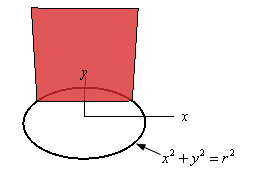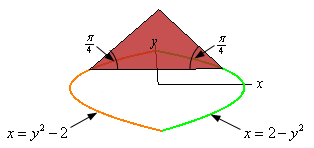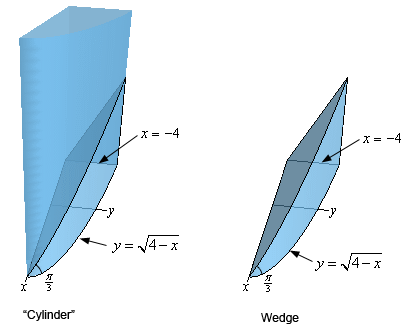Paul's Online Notes
Home / Calculus I / Applications of Integrals / More Volume Problems
Show Mobile Notice Show All Notes Hide All Notes
Mobile Notice
You appear to be on a device with a "narrow" screen width (i.e. you are probably on a mobile phone). Due to the nature of the mathematics on this site it is best views in landscape mode. If your device is not in landscape mode many of the equations will run off the side of your device (should be able to scroll to see them) and some of the menu items will be cut off due to the narrow screen width.

### Section 6.5 : More Volume Problems

1. Find the volume of a pyramid of height $$h$$ whose base is an equilateral triangle of length $$L$$. Solution
2. Find the volume of the solid whose base is a disk of radius $$r$$ and whose cross-sections are squares. See figure below to see a sketch of the cross-sections.Solution
3. Find the volume of the solid whose base is the region bounded by $$x = 2 - {y^2}$$ and $$x = {y^2} - 2$$ and whose cross-sections are isosceles triangles with the base perpendicular to the $$y$$-axis and the angle between the base and the two sides of equal length is $$\frac{\pi }{4}$$. See figure below to see a sketch of the cross-sections.Solution
4. Find the volume of a wedge cut out of a “cylinder” whose base is the region bounded by $$y = \sqrt {4 - x}$$, $$x = - 4$$ and the $$x$$-axis. The angle between the top and bottom of the wedge is $$\frac{\pi }{3}$$. See the figure below for a sketch of the “cylinder” and the wedge (the positive $$x$$-axis and positive $$y$$-axis are shown in the sketch – they are just in a different orientation).Solution